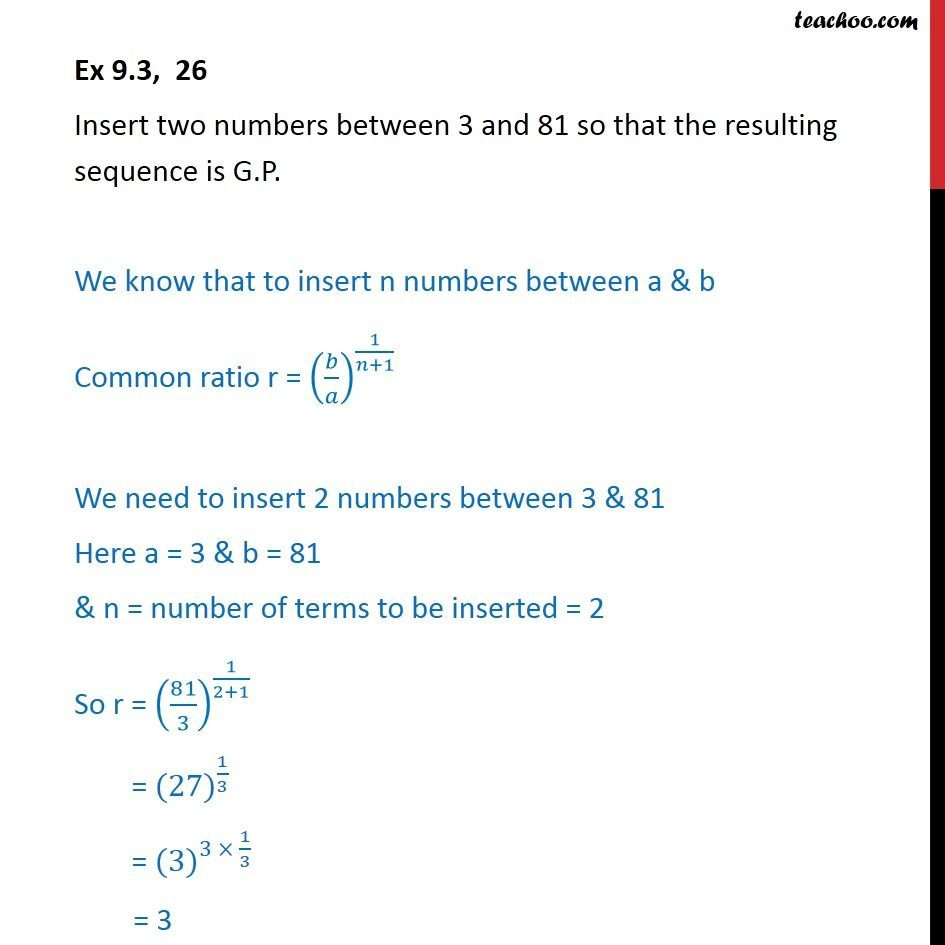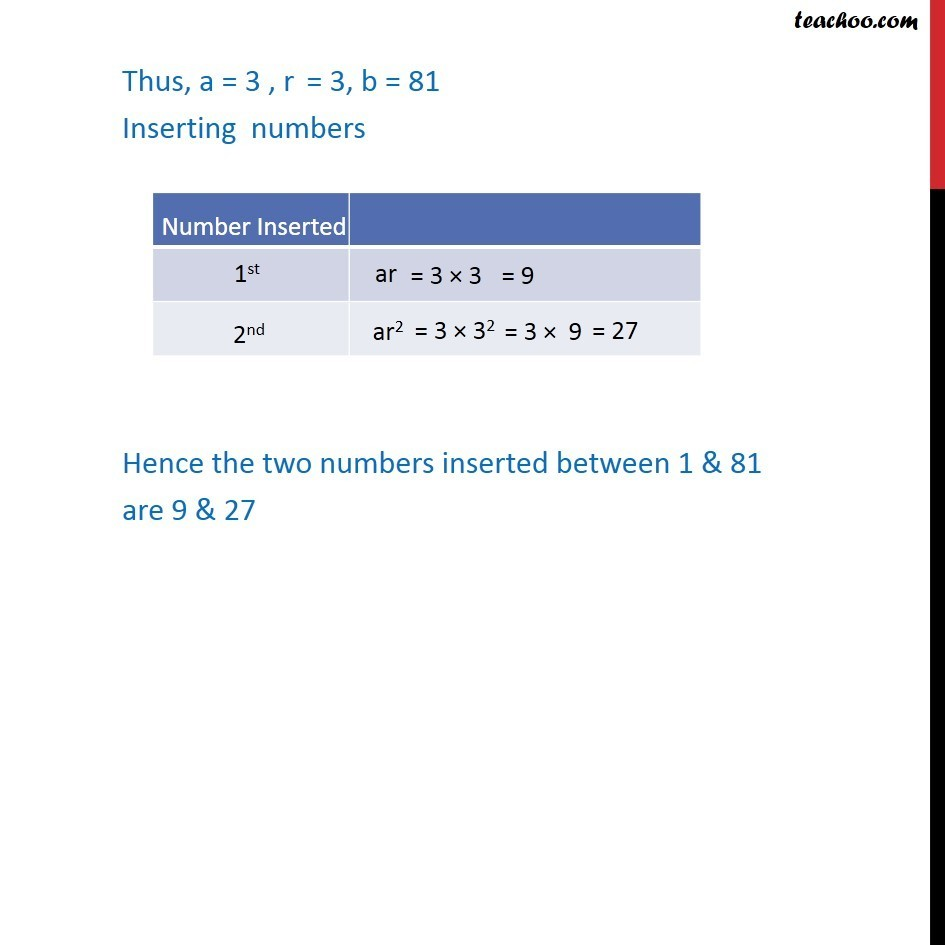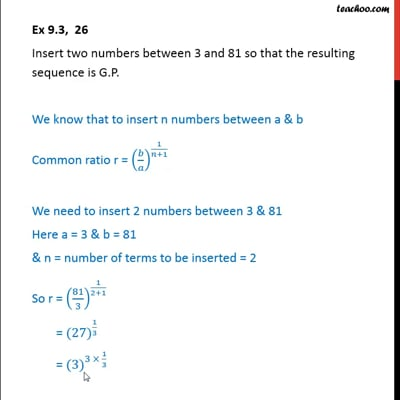Ex 9.3

Chapter 9 Class 11 Sequences and Series (Term 1)
Serial order wiseThis video is only available for Teachoo black users

### Transcript

Ex 9.3, 26 Insert two numbers between 3 and 81 so that the resulting sequence is G.P. We know that to insert n numbers between a & b Common ratio r = ( / )^(1/( +1)) We need to insert 2 numbers between 3 & 81 Here a = 3 & b = 81 & n = number of terms to be inserted = 2 So r = (81/3)^(1/(2+1)) = (27) ^(1/3) = (3) ^(3 1/3 ) = 3 Ex 9.3, 26 Insert two numbers between 3 and 81 so that the resulting sequence is G.P. We know that to insert n numbers between a & b Common ratio r = ( / )^(1/( +1)) We need to insert 2 numbers between 3 & 81 Here a = 3 & b = 81 & n = number of terms to be inserted = 2 So r = (81/3)^(1/(2+1)) = (27) ^(1/3) = (3) ^(3 1/3 ) = 3 Thus, a = 3 , r = 3, b = 81 Inserting numbers Hence the two numbers inserted between 1 & 81 are 9 & 27 Thus, a = 3 , r = 3, b = 81 Inserting numbers Hence the two numbers inserted between 1 & 81 are 9 & 27